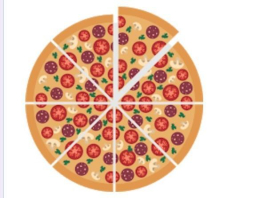# Two boxes 3

Two boxes of pizza of the same size are left on the table. One box has 1/2 of a pizza, and the other has 3/4 of a pizza left in it. Then, Mother divides all the pizzas into 1/8 each. How many were many slices of pizza left?

n =  10

### Step-by-step explanation:Did you find an error or inaccuracy? Feel free to write us. Thank you!

Tips for related online calculators
Need help calculating sum, simplifying, or multiplying fractions? Try our fraction calculator.